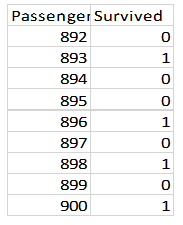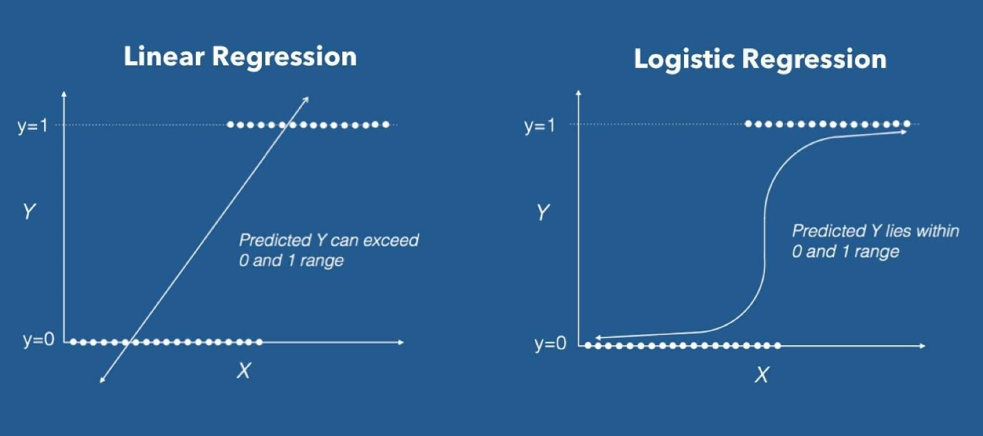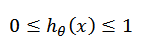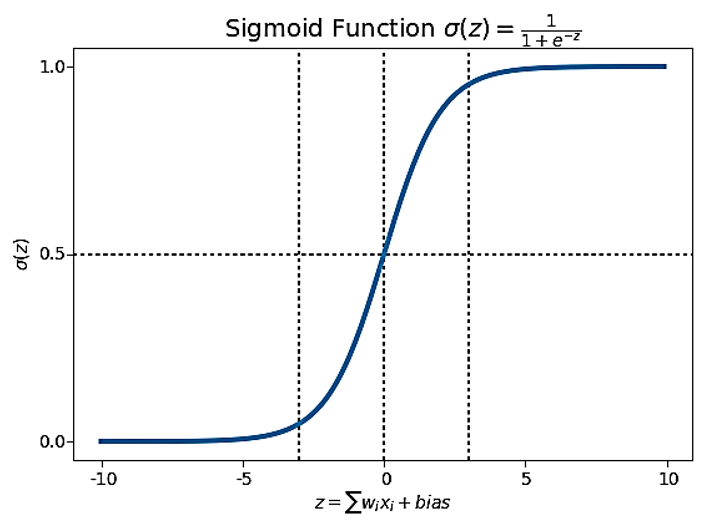### Logistic Regression in Machine Learning

• date 15th February, 2020 |
• by Prwatech |

# Logistic Regression in Machine Learning

Logistic Regression in Machine Learning, Are you the one who is looking forward to knowing logistic regression introduction in Machine Learning? Or the one who is looking forward to know types of logistic regression models and assumptions for Logistic Regression or Are you dreaming to become to certified Pro Machine Learning Engineer or Data Scientist, then stop just dreaming, get your Data Science certification course with Machine Learning from India’s Leading Data Science training institute.

Logistic Regression is one of popular Machine Algorithm which is used for predicting dependent variable using set of independent variables. This will perform well for all wide range of problems. In this blog, we will learn about types of Logistic regression models and what is sigmoid function along with logistic regression example.Do you want to know Assumptions for Logistic Regression, So follow the below mentioned logistic regression in machine learning with examples from Prwatech and take advanced Data Science training with Machine Learning like a pro from today itself under 10+ Years of hands-on experienced Professionals.

## Logistic Regression Introduction

Logistic regression is the type of regression predictive analysis which associates a functional bonding between categorical dependent variable and independent variable or variables on basis of estimation of probabilities. i.e. the dependent variable will be a categorical data.

Some common examples of classification problems are

Will it rain or won’t.

Online transactions are Fraud or not Fraud.

Is movie good or not.

Logistic regression the output is transformed using the logistic sigmoid function to return a probability value.

## Types of Logistic Regression Models

Binary Logistics Regression
Multi-Linear Logistic Regression

### Binary Logistic Regression

Here the Dependent column with have only two values i.e. true or false, 0 or 1.

Ex) In Titanic Ship Accident passenger survived or not survived### Multi Linear Logistic Regression

Here the Dependent variable column will contain more than two values but in the repeated format.

Ex) The Outlook can be sunny, cloudy or overcast

 Temperature      (in  ̊ C) Humidity Wind speed Outlook 40 Hot 6.0 Sunny 43 Hot 11.1 Sunny 36 Hot 9.3 Overcast 39 Humidity 8.1 CloudyLogistic Regression uses Sigmoid function also known as logistic function for its calculation. The hypothesis of logistic regression makes to limit the cost function between the limit 0 and 1.

As a result, linear functions fail to represent it as it can have a value more than 1 or less than 0 and it is impossible as per the hypothesis of logistic regression.## What is Sigmoid Function?

For mapping predicted values to probabilities, we implement Sigmoid function. The function maps any real value into another value between 0 and 1. In machine learning, we use sigmoid to map predictions to probabilities.## Assumptions for Logistic Regression

Data is free from missing values

The Predicted variable must be categorical variable with order values.

All the predictors are independent of each other

There are at least 50 observations per predictor variable to ensure reliable results.

## Logistic Regression Example

While applying logistic regression analysis on dataset we have to follow the steps:

### Import all the Libraries:

import numpy as np

import pandas as pd

from sklearn import metrics

### Divided the dataset into dependent and independent

X=data.drop([‘Outcome’],axis=1)

Y=data[‘Outcome’]

### Checking of missing values.

data.isnull.sum()

### Divide the dataset for training an testing

from sklearn.model_selection import train_test_split

from sklearn.linear_model import LogisticRegression

X_train, X_test, y_train, y_test = train_test_split(X, Y, test_size=0.3, random_state=0)

### Implement the logistic model

reg = LogisticRegression()

reg.fit(X_train, y_train)

### Determine predicted value of dependent variable.

y_pred = reg.predict(X_test)

from sklearn import datasets, linear_model, metrics

print(“Logistic Regression model accuracy(in %):”,

metrics.accuracy_score(y_test, y_pred)*100)

Result:

Logistic Regression model accuracy (in%): 78.35

(Note : Result for different data set can be different.)

We hope you understand logistic regression in machine learning concepts and types of logistic regression models.Get success in your career as a Data Scientist by being a part of the Prwatech, India’s leading Data Science training institute in Bangalore.

### Quick Support